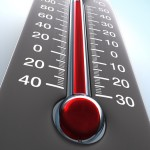Temperature Conversion

Temperature Conversion helps you convert the values ​​of the three temperature scales commonly used in the world.

Fahrenheit:
Celsius:
Kelvin:

Temperature Conversion Formula – About Temperature

Temperature is a physical property that expresses, in quantity, the general concept of hot. Matters with few degrees of higher, or many degrees of negative temperature are cold, at the same time as degrees of higher temperatures are referred to as hot. It is measured with thermometers, which can be calibrate, after the temperature conversion, to a variety of scales.

Celsius scale (°C) is standard in most of the world for the majority temperature measurements. A small number of countries, like the United States, use the Fahrenheit scale for general purposes, a scale on which water freezes at 32 °F and boils at 212 °F.

The International System of Units defines a scale for the temperature by using the reproducible temperature of the triple point of water as a second reference point. This triple point is set at 273.16 units of the measurement increment, which has been named the Kelvin.

Temperature Conversion Formula

Use these formulas for temperature conversionCelsius | Fahrenheit | Kelvin

F = C . 9/5 + 32

Temperature Conversion – Fahrenheit to Celsius:

C = (F-32).5/9

Temperature Conversion – Celsius to Kelvin: C + 273 =K

Kelvin to Celsius:

K – 273 = C

Fahrenheit to Kelvin:

Kelvin
KelvinF = K = 5/9 ( F – 32) + 273

Kelvin to Fahrenheit:

K = 5/9 ( F – 32) + 273